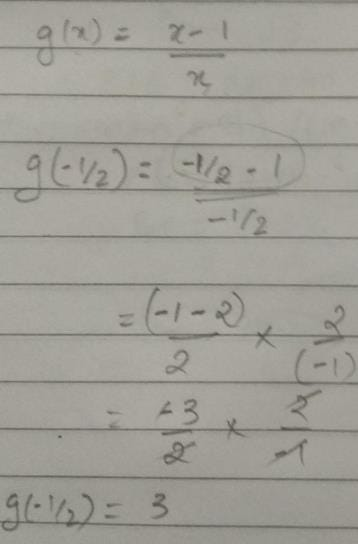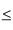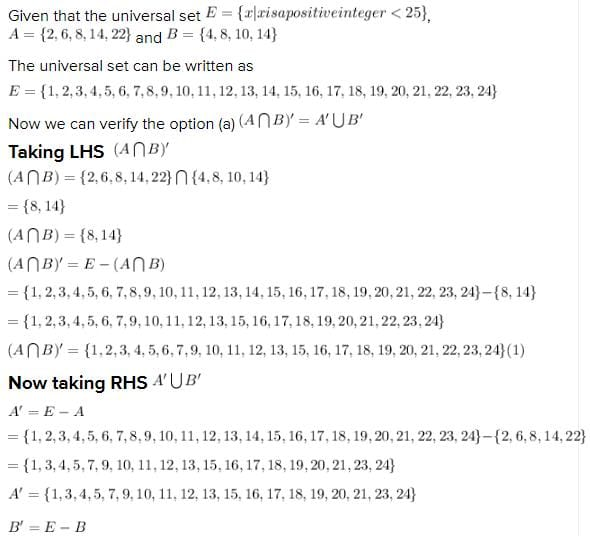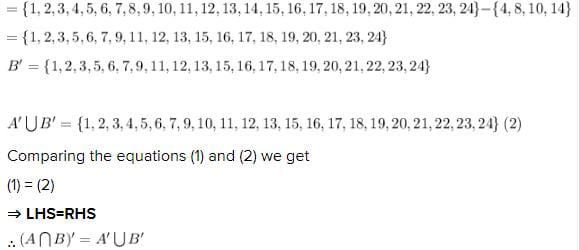# Test: Sets- 2

## 40 Questions MCQ Test Quantitative Aptitude for CA CPT | Test: Sets- 2

Description
Attempt Test: Sets- 2 | 40 questions in 40 minutes | Mock test for CA Foundation preparation | Free important questions MCQ to study Quantitative Aptitude for CA CPT for CA Foundation Exam | Download free PDF with solutions
QUESTION: 1

Solution:
QUESTION: 2

### If g(x) = (x–1)/x, g(–½) is

Solution:QUESTION: 3

### If f(x) = 1/1–x and g(x) = (x–1)/x, than fog(x) is

Solution:
QUESTION: 4

If f(x) = 1/1–x and g(x) = (x–1)/x, then g of(x) is

Solution:
QUESTION: 5

The function f(x) = 2x is

Solution:
QUESTION: 6

The range of the function f(x) = log10(1 + x) for the domain of real values of x when 0 ≤ x ≤9 is

Solution:
QUESTION: 7

The Inverse function f–1 of f(x) = 2x is

Solution:
QUESTION: 8

If f(x) = x+3, g(x) = x2, then fog(x) is

Solution:
QUESTION: 9

If f(x) = x+3, g(x) = x2 then f(x).g(x) is

Solution:
QUESTION: 10

The Inverse h–1 when h(x) = log10x is

Solution:
QUESTION: 11

For the function h(x) = 101+x the domain of real values of x where 0x9 , the range is

Solution:
QUESTION: 12

"Is smaller than" over the set of eggs in a box is

Solution:
*Multiple options can be correct
QUESTION: 13

"Is equal to" over the set of all rational numbers is

Solution:
*Multiple options can be correct
QUESTION: 14

"has the same father as" …… over the set of children

Solution:
QUESTION: 15

"is perpendicular to " over the set of straight lines in a given plane is

Solution:
QUESTION: 16

"is the reciprocal of" …….. over the set of non-zero real numbers is

Solution:
*Multiple options can be correct
QUESTION: 17

{(x, y)/x ∈ ×, y ∈ y, y = x} is

Solution:
*Multiple options can be correct
QUESTION: 18

{(x,y)/x + y = 2x where x and y are positive integers}, is

Solution:
QUESTION: 19

"Is the square of" over n set of real numbers is

Solution:
QUESTION: 20

If A has 32 elements, B has 42 elements and AUB has 62 elements, the number of elements in A ∩ B is

Solution:
QUESTION: 21

In a group of 20 children, 8 drink tea but not coffee and 13 like tea. The number of children drinking coffee but not tea is

Solution:
QUESTION: 22

The number of subsets of the sets {6, 8, 11} is

Solution:
QUESTION: 23

The sets V = {x / x+2=0}, R={x / x2+2x=0} and S = {x : x2+x–2=0} are equal to one another if x is equal to

Solution:
QUESTION: 24

If the universal set E = {x |x is a positive integer <25} , A = {2, 6, 8, 14, 22}, B = {4, 8, 10, 14} then

Solution:QUESTION: 25

If the set P has 3 elements, Q four and R two then the set P×Q×R contains

Solution:
QUESTION: 26

Given A = {2, 3}, B = {4, 5}, C = {5, 6} then A × (B ∩ C) is

Solution:
QUESTION: 27

A town has a total population of 50,000. Out of it 28,000 read the newspaper X and 23000 read Y while 4000 read both the papers. The number of persons not reading X and Y both is

Solution:
QUESTION: 28

If A = { 1, 2, 3, 5, 7} and B = {1, 3, 6, 10, 15}. Cardinal number of A~B is

Solution:
QUESTION: 29

Which of the diagram is graph of a function

Solution:
QUESTION: 30

At a certain conference of 100 people there are 29 Indian women and 23 Indian men. Out of these Indian people 4 are doctors and 24 are either men or doctors. There are no foreign doctors. The number of women doctors attending the conference is

Solution:
QUESTION: 31

Let A = {a, b}. Set of subsets of A is called power set of A denoted by P(A). Now n(P(A) is

Solution:
QUESTION: 32

Out of 2000 employees in an office 48% preferred Coffee (c), 54% liked (T), 64% used to smoke (S). Out of the total 28% used C and T, 32% used T and S and 30% preferred C and S, only 6% did none of these. The number having all the three is

Solution:

Total = 2000  = 100 %

C = 48 %

T = 54 %

S = 64 %

C ∩ T = 28 %

T ∩ S = 32%

C ∩ S = 30 %

Having all three = C ∩ T ∩ S  = ?

None = 6 %

Total = C + T + S - C ∩ T  - T ∩ S - C ∩ S + C ∩ T ∩ S + None

=> 100 = 48 + 54 + 64 - 28 - 32 - 30 + C ∩ T ∩ S + 6

=> C ∩ T ∩ S = 18 %

18 % of 2000 = (18/100) * 2000 = 360

360 having all the three

QUESTION: 33

Referred to the data of

Out of 2000 employees in an office 48% preferred Coffee (c), 54% liked (T), 64% used to smoke (S). Out of the total 28% used C and T, 32% used T and S and 30% preferred C and S, only 6% did none of these. The number having all the three is

Q. The number of employees having T and S but not C is

Solution:
QUESTION: 34

Referred to the data of

Out of 2000 employees in an office 48% preferred Coffee (c), 54% liked (T), 64% used to smoke (S). Out of the total 28% used C and T, 32% used T and S and 30% preferred C and S, only 6% did none of these. The number having all the three is

Q. The number of employees preferring only coffee is

Solution:
QUESTION: 35

If f(x) = x+3, g(x) = x2, then gof(x) is

Solution:
QUESTION: 36

If f(x) = 1/1–x, then f -1(x) is

Solution:
QUESTION: 37

Following set notations represent: – A ⊂ B; x∉ A; A ⊃B; {0}; A ⊄B

Solution:
QUESTION: 38

Represent the following sets in set notation: – Set of all alphabets in English language set of all odd integers less than 25 set of all odd integers set of positive integers x satisfying the equation x2 +5x+7=0 : -

Solution:
QUESTION: 39

Re-write the following sets in a set builder form: - A={a, e, i, o, u} B={1, 2, 3, 4 ….} C is a set of integers between –15 and 15.

Solution:
QUESTION: 40

If V={0, 1, 2, …9}, X={0, 2, 4, 6, 8}, Y={3, 5, 7} and Z={3 7} then Y ∪ Z, (V ∪ Y) ∩ X, (X ∪ Z) ∪ V  are respectively: –

Solution:Use Code STAYHOME200 and get INR 200 additional OFF Use Coupon Code